# JP3989261B2 - Digital camera - Google Patents

## Info

Publication number
JP3989261B2
JP3989261B2 JP2002042205A JP2002042205A JP3989261B2 JP 3989261 B2 JP3989261 B2 JP 3989261B2 JP 2002042205 A JP2002042205 A JP 2002042205A JP 2002042205 A JP2002042205 A JP 2002042205A JP 3989261 B2 JP3989261 B2 JP 3989261B2
Authority
JP
Japan
Prior art keywords
lens
af
position
means
step
Prior art date
Legal status (The legal status is an assumption and is not a legal conclusion. Google has not performed a legal analysis and makes no representation as to the accuracy of the status listed.)
Expired - Fee Related
Application number
JP2002042205A
Other languages
Japanese (ja)
Other versions
JP2003244519A (en
Inventor

Original Assignee
オリンパス株式会社
Priority date (The priority date is an assumption and is not a legal conclusion. Google has not performed a legal analysis and makes no representation as to the accuracy of the date listed.)
Filing date
Publication date
Application filed by オリンパス株式会社 filed Critical オリンパス株式会社
Priority to JP2002042205A priority Critical patent/JP3989261B2/en
Publication of JP2003244519A publication Critical patent/JP2003244519A/en
Application granted granted Critical
Publication of JP3989261B2 publication Critical patent/JP3989261B2/en
Expired - Fee Related legal-status Critical Current
Anticipated expiration legal-status Critical

• 238000003384 imaging method Methods 0.000 claims description 59
• 230000000875 corresponding Effects 0.000 claims description 19
• 230000001276 controlling effects Effects 0.000 claims description 16
• 238000000034 methods Methods 0.000 description 34
• 230000000694 effects Effects 0.000 description 1
• 239000000463 materials Substances 0.000 description 1

## Images

•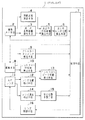•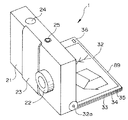•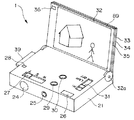•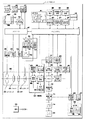•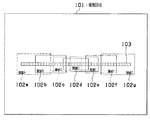•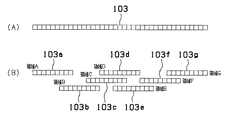•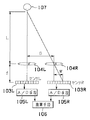•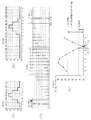•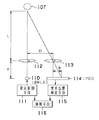•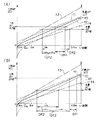•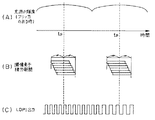•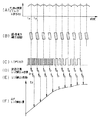•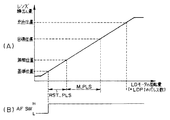•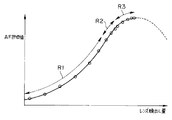•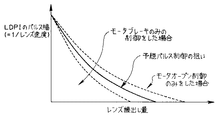•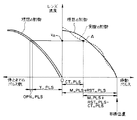•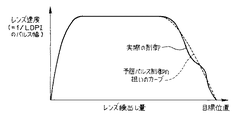•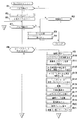•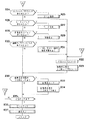•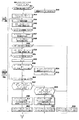•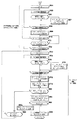•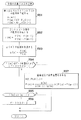•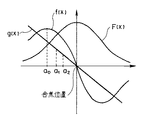•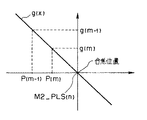•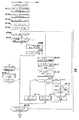•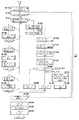## Description


BACKGROUND OF THE INVENTION
The present invention relates to a digital camera, and more particularly to a digital camera that captures and digitizes a subject image.

[Prior art]
In a digital camera that captures a subject image with an image sensor, digitizes the obtained image signal, performs image processing, etc., and records the image on a recording medium or the like, the subject image formed on the image sensor is focused. Therefore, various methods are employed.

Japanese Patent Laid-Open No. 6-62304 as an example of such a technique includes an extraction unit that extracts a sharp signal from a video signal, and a lens position detection unit that detects a lens position, and a focus lens position; A technique for controlling the focus lens driving means based on the sharpness signal and the delay information of the sharpness signal is described. In this publication, the past focus lens position is stored in consideration of the delay time until the sharpness signal is input to the control device, and the peak of the contrast amount is obtained by using the stored lens position. This is so-called hill-climbing AF, in which the motor is rotated in reverse and the focus is adjusted.

[Problems to be solved by the invention]
However, in the one described in Japanese Patent Laid-Open No. 6-62304, the delay time is taken into consideration so as to prevent the deviation between the lens position and the sharp signal, but the reason is the hill-climbing AF. In addition, it will go back and forth until it reaches the in-focus position which is the peak of the contrast amount, and it takes time to perform forward / reverse rotation of the motor and to correct the backlash, It takes time to stop the lens at the in-focus position.

The present invention has been made in view of the above circumstances, and an object thereof is to provide a digital camera capable of performing an accurate AF operation at a higher speed.

[Means for Solving the Problems]
In order to achieve the above object, a digital camera according to a first invention includes an auxiliary AF unit for detecting a subject distance, and an imaging unit for photoelectrically converting a subject image and integrating it for a predetermined integration period and then outputting it as an image signal. And an AF lens for focusing the subject image formed on the imaging unit, and a contrast evaluation value of the subject image formed on the imaging unit based on an image signal output from the imaging unit Contrast evaluation value calculating means, lens position detecting means for detecting the lens position of the AF lens, lens control means for controlling and moving the AF lens based on the lens position detected by the lens position detecting means, A plurality of lens positions detected by the lens position detecting means in accordance with the movement of the AF lens by the lens control means. And the plurality of contrast evaluation values calculated by the contrast evaluation value calculation unit corresponding to each of the plurality of lens positions, and the lens position at which the contrast evaluation value of the subject image formed on the imaging unit peaks Contrast peak position calculating means for calculating the image, and corresponding to the subject distance detected by the auxiliary AF means When the lens position is determined from the far side of the subject distance, the far side in the in-focus area is used to asymptotically approach the focus position without passing the focus position. First lens position As Calculation And While performing the first lens extension for the AF lens with the first lens position as a target, and during the first lens extension, the calculation of the contrast evaluation value of the image and the calculation of the contrast evaluation value are performed. The lens position is detected multiple times, and these Multiple times Based on the calculation result and the detection result, the contrast peak position calculation means The contrast evaluation value is based on at least two sets of the contrast evaluation value calculated or detected a plurality of times during the first lens extension and the lens position at the time of calculating the contrast evaluation value. Based on the result obtained by approximating the change in the contrast evaluation value with respect to the lens position with a normal distribution in the vicinity where the peak is, and dividing the normal distribution differentiated by this normal distribution, Contrast evaluation value peaks In focus position The second lens position is calculated, and the second lens is extended to the AF lens with the second lens position as a target.

A digital camera according to a second aspect of the invention is the digital camera according to the first aspect of the invention, wherein the AF speed is detected from the lens speed detecting means for detecting the moving speed of the AF lens, and the moving speed detected by the lens speed detecting means. Stop position prediction means for predicting the stop position of the lens, and the lens control means controls the AF lens based on the difference between the target extension position and the expected stop position.

Further, the digital camera according to a third aspect of the invention is the digital camera according to the first aspect of the invention, wherein the auxiliary AF means detects the subject distance by phase difference AF, and detects the two images. The first focus distance calculation means for obtaining the first focus distance based on the phase of the two images detected by the detection means, and the measurement based on the undulations of the two images detected by the detection means. A distance measurement error calculating means for calculating a distance error, and a second focus distance with a small amount of feeding within a range where there is a possibility of focusing based on the first focus distance and the distance measurement error. Second focus distance calculation means, and the first lens position is obtained based on the second focus distance.

DETAILED DESCRIPTION OF THE INVENTION
Embodiments of the present invention will be described below with reference to the drawings.
1 to 26 show an embodiment of the present invention, and FIG. 1 is a block diagram showing a conceptual configuration of a digital camera.

The digital camera 1 includes, for example, an auxiliary AF unit 2 that detects a subject distance by phase difference AF, and a first focusing distance that is obtained based on the phases of two images detected by the auxiliary AF unit 2. Focus distance calculation means 3, distance measurement error calculation means 4 for calculating a distance measurement error based on the undulation of the two images, and focusing distance based on the first focus distance and the distance measurement error. There is a possibility that the second focus distance calculation means 5 for obtaining the second focus distance with a small amount of feeding and the second focus distance calculated by the second focus distance calculation means 5 are used. First lens position calculating means 6 for calculating a first lens position for extending a lens, which will be described later, an imaging means 8 for photoelectrically converting a subject image and outputting an image signal, and detected by the imaging means 8 Periodic flicker from subject brightness changes A flicker timing detection means 9 that serves as both a flicker detection means and a stability timing detection means for detecting a stable timing that can be detected stably without being affected by the flicker, and the imaging means 8 A contrast evaluation value calculating unit 10 that calculates a contrast evaluation value (specifically, a contrast amount) of a subject image formed on the imaging unit 8 based on an output image signal, and a subject image on the imaging unit 8. The AF lens 11 is moved by an AF lens 11 for focusing in the photographing optical system for forming an image, a lens position detecting means 12 for detecting the lens position of the AF lens 11, and a lens control means 16 described later. And a plurality of lens positions detected by the lens position detecting means 12 according to A contrast for calculating a lens position at which the contrast amount of the subject image formed on the imaging means 8 peaks based on a plurality of contrast evaluation values calculated by the contrast evaluation value calculation means 10 corresponding to each position. Peak position calculating means 13, lens speed detecting means 14 for detecting the moving speed of the AF lens 11, and stop position prediction for predicting the stopping position of the AF lens 11 from the moving speed detected by the lens speed detecting means 14. The AF lens 11 is controlled on the basis of the difference between the stop position predicted by the means 15 and the stop position predicted by the stop position predicting means 15 and the extension amount from the lens position detected by the lens position detection means 12 to the target extension position. The lens control means 16 to be moved and the digital camera 1 including the elements as described above are integrated. And control means 7 for comprehensive control.

The operation of the digital camera 1 configured in this way is as follows.

First, the first lens position is obtained by the auxiliary AF means 2, and the first lens is extended with the first lens position as a target.

While the first lens is being extended, the contrast evaluation value calculation means 10 detects the contrast amount of the image and the lens position detection means 12 detects the current lens position a plurality of times.

Then, based on these detection results, the second lens position where the contrast amount is maximized is calculated, and the second lens is extended with the second lens position as a target.

When the AF lens 11 is moved, the stop position predicting means 15 calculates the expected stop position based on the lens speed detected by the lens speed detecting means 14, and the lens control means 16 determines the target feeding position. This is based on the difference from the expected stop position.

More specifically, the first lens position is obtained as follows.

The auxiliary AF means 2 is configured to be able to perform distance measurement on a plurality of distance measurement areas. The auxiliary AF means 2 performs distance measurement on the plurality of distance measurement areas, and is the first closest to the distance measurement area. A distance measurement error is calculated for the distance measurement area where the in-focus distance is obtained, and a second in-focus distance that is a distance with a small amount of feeding out of a distance that is likely to be in focus, in consideration of the distance measurement error. Ask for. Then, based on the second focus distance, a first lens position for performing the first extension is calculated.

First, the AF lens 11 is extended with the first lens position as a target. While the AF lens 11 is being extended to the first lens position, contrast AF is performed on the distance measuring area that is closest to the auxiliary AF means 2 to provide a more accurate in-focus position. The position is calculated, and thereafter, the second lens position is set as a target and the feeding is performed.

The imaging unit 8 is also configured to be able to image a plurality of ranging areas corresponding to the plurality of ranging areas by the auxiliary AF unit 2.

In general, there is a backlash in the lens payout mechanism, and when the payout is carried out after the payout, it is necessary to remove the backlash by moving more than the backlash. In addition, when changing the direction of lens movement, both braking time and return time are required because the brake must be braked to stop and then controlled to change direction. It will take a long time to reach the focus.

On the other hand, since the first lens position is calculated based on the distance with a small amount of extension within the focusable range in this way, the direction can be changed without being overdrawn from the true focus position. It is possible to control the lens position without changing, and it is possible to focus at high speed without the need for backlash removal.

The auxiliary AF means 2 may be provided with a dedicated element, but it is also possible to use an area other than the imaging area of the imaging means 8. As the AF method of the auxiliary AF means, phase difference AF and active AF can be used as will be described later, and other various means can also be used.

Further, the calculation of the position where the contrast amount of the subject image formed on the image pickup unit 8 reaches a peak (that is, the in-focus position) is performed in a normal distribution or the like, as will be described in detail later. It is calculated by approximation.

Since the contrast amount peak position is a position where the contrast change amount becomes zero, the contrast amount is detected by performing extrapolation by performing the operation of detecting the contrast amount a plurality of times while the AF lens 11 is extended.

Further, when performing contrast AF, when the imaging unit 8 performs integration for the selected distance measurement area, control is performed so that the intermediate timing of the integration period is a timing with less flicker change, and further, The lens position of the AF lens 11 is also detected at this intermediate timing. Thereby, it is possible to suppress fluctuations in luminance due to flicker.

Next, FIG. 2 is a perspective view showing the appearance of the digital camera 1 from the lens side, and FIG. 3 is a perspective view showing the appearance when the digital camera 1 is used as a PDA (Personal Digital (Data) Assistants). In FIG. 3, the lens side is arranged downward.

In the digital camera 1, a lens barrel 22 containing a lens that is a zoom optical system is disposed on the front side of a main body 21, and the lens barrel 22 is opened as the barrier 23 is opened. It protrudes from the main body 21 and is driven to the wide end where photographing is possible. When the barrier 23 is opened and closed, a barrier switch (not shown) is turned on / off accordingly. That is, when the barrier 23 is opened, the digital camera 1 operates as a camera.

Also, on the upper surface of the digital camera 1, a release switch 24 for inputting a photographing operation and a PDA power for turning on the power for functioning the digital camera 1 as a PDA in a state where the barrier 23 is closed. A switch 25 is provided.

Further, on the back side of the main body 21 of the digital camera 1, a finder 26 for confirming a subject at the time of photographing, a zoom switch 27 for changing the focal length of the lens, and the luminance of a sheet-like light source 33 described later. A brightness setting volume 31 for adjusting the image quality, and each member used when the digital camera 1 operates as a PDA as described later, that is, an image inversion switch 29, an image display mode switch 30, and a magnet 28. It is arranged.

The digital camera 1 is provided with a display unit 89 that can be opened and closed by a hinge 32 a with respect to the back side of the main body 21. The display unit 89 is formed in a thin plate shape having a size that exposes the finder 26 and the zoom switch 27 when the display unit 89 is in a closed position substantially along the back side of the main body 21, and is transmissive for displaying an image. LCD 35, a sheet-like light source 33 for irradiating the LCD 35 with illumination light from the back side, and a diffusion plate 34 for uniformly diffusing the light emitted from the sheet-like light source 33 to the LCD 35 Are stacked and held in a support frame 32 having a rectangular frame shape.

The display unit 89 constitutes an image display member 86 (see FIG. 4) described later by the LCD 35, the diffusion plate 34, and the sheet-like light source 33, and further has a touch panel 88 (see FIG. 4). Information can be input via the touch panel 88.

In the above description, the diffusion plate 34 and the sheet-like light source 33 are used. However, instead of these, for example, an organic EL sheet or the like may be used.

As described above, since the rectangular frame shape is used as the support frame 32, if each member constituting the image display member 86 and the touch panel 88 is made of a material having translucency, the outer side from the back side is used. Light can be used as a light source. As a result, when the outside light is bright, the brightness setting volume 31 is operated to turn the brightness low or off, thereby clearing the image while suppressing the battery consumption. It becomes possible to observe.

A magnet 36 is fitted into, for example, the upper left corner of the support frame 32. When the display unit 89 is closed, the magnet 28 is attracted to the rear surface of the main body 21 and is not prepared. Further, the display unit 89 is prevented from being opened, and is easily opened when a rotational force is applied by hand or the like.

The digital camera 1 can be detachably mounted with a memory card 39 for storing captured image data.

FIG. 4 is a block diagram mainly showing an electrical configuration of the digital camera 1.

The digital camera 1 includes a zoom lens 44, a shutter 45, an aperture 46, and an AF lens 47. The lens barrel 22, zoom lens control means 48 that controls and moves the zoom lens 44, and the shutter 45. Shutter control means 49 for controlling and driving the diaphragm, diaphragm control means 50 for controlling and driving the diaphragm 46, an LD motor 54 serving as a drive source for moving the AF lens 47, and controlling the LD motor 54 A focus lens control circuit 51 including a focus motor drive circuit 52 for driving the focus lens and a focus lens position detection circuit 53 for detecting the lens position of the AF lens 47; lenses 56R and 56L for forming a plurality of images; Sensors as detection means for converting a plurality of images formed by the lenses 56R and 56L into electrical signals 7, a phase difference AF means 58 for performing phase difference AF based on an output from the sensor unit 55, a strobe light emitting unit 59 that irradiates illumination light toward the subject, and a light emission power to the strobe light emitting unit 59. The strobe main capacitor 60 to be supplied, and the strobe charging for controlling the light emission by controlling the charging to the strobe main capacitor 60 and the discharging to the strobe light emitting unit 59 based on the output of the sub CPU 42 and the phase difference AF means 58 described later. Acquire information as necessary from the light emission control means 61, the zoom lens control means 48, the shutter control means 49, the aperture control means 50, the focus lens control means 51, the phase difference AF means 58, and the strobe charge light emission control means 61. While controlling the sub CPU 42, the zoom lens 44 and the AF lens 47, An image sensor 65 that photoelectrically converts a subject image to be imaged and outputs an image signal; an image area specifying means 66 for specifying an image area in the image sensor 65; and an analog output from the image sensor 65 An A / D circuit 67 for converting the image signal into digital image data, a signal level control means 68 for adjusting the gain of the image sensor 65 based on the output of the A / D circuit 67, and the A / D circuit 67, a memory 71 for storing image data output from 67, an image processing circuit 72 for performing image processing on the image data stored in the memory 71, and an image compression means for compressing the image data processed by the image processing circuit 72. 73, a memory I / F 74 for recording the image data compressed by the image compression means 73 on the memory card 39, and a control of the memory I / F 74 The memory card 39 for storing image data, the luminance detecting means 76 as a subject luminance detecting means for reading out the image data stored in the memory 71 and detecting the luminance of the subject, and the luminance detecting means 76 Flicker timing detection means 77 for detecting a flicker based on the detected luminance and detecting a timing not affected by the flicker, and an image pick-up by the image sensor 65 based on the timing detected by the flicker timing detection means 77 A timing generation circuit 69 that performs digitization by the A / D circuit 67, a contrast evaluation value calculation unit 78 that reads out image data stored in the memory 71 and calculates a contrast evaluation value (for example, a contrast amount), and the contrast In the output of the evaluation value calculation means 78 The contrast evaluation value differentiating means 79 for calculating the differentiation of the contrast evaluation value, the focus position predicting means 80 for predicting the focus position based on the output of the contrast evaluation value differentiating means 79, and the contrast evaluation value calculating means 78. Based on the output, for example, a focus detection unit 81 that performs focus detection by performing hill-climbing AF, and an image that controls the image display member 86 described above to display the image data output through the image compression unit 73. A display comprising display control means 85, an image display member 86 that is controlled by the image display control means 85 to display images and characters, and a touch panel 88 arranged along the display surface of the image display member 86. Unit 89, touch panel input means 87 that generates and outputs an input signal based on the output from touch panel 88, and Operation means including a barrier switch for turning on / off the power of the digital camera 1 in conjunction with an operation of opening and closing the barrier 23, the release switch 24, the zoom switch 27, the PDA power switch 25, a mode switch, and the like. 91, a USB (Universal Serial Bus) terminal 92, a USB control means 93 for controlling the USB terminal 92, and an IEEE802. b circuit 94 and the IEEE802. b IEEE802 for controlling the circuit 94. b control means 95, a Bluetooth circuit 96 for connecting devices wirelessly, a Bluetooth control means 97 for controlling the Bluetooth circuit 96, and a processing program for operating a main CPU 41, which will be described later, and this digital camera 1 to control various circuits connected to the sub-CPU 42 by communicating with the sub-CPU 42 and connected to itself. And a main CPU 41 for controlling the entire digital camera 1 including each circuit.

The image pickup circuit 64 is configured by the image pickup element 65, the image pickup area specifying means 66, the A / D circuit 67, the signal level control means 68, the timing generation circuit 69, and the like.

The memory card 39 can be attached to and detached from the digital camera 1, and the battery 99 can be exchanged, for example.

In the configuration shown in FIG. 4, the sensor unit 55 and the phase difference AF means 58 are the auxiliary AF means 2 and the main CPU 41 is the first focusing distance calculation means 3, The error calculating unit 4, the second focusing distance calculating unit 5, the first lens position calculating unit 6, the control unit 7, the stop position predicting unit 15, the imaging element 65 in the imaging unit 8, and the flicker timing detecting unit 77. In the flicker timing detection means 9, the contrast evaluation value calculation means 78 is in the contrast evaluation value calculation means 10, the AF lens 47 is in the AF lens 11, and the focus lens position detection circuit 53 is in the lens position detection means 12 and the lens speed detection means 14. The focus position predicting means 80 is used for the contrast peak position calculating means 13, and the focus motor driving circuit 52 is used for the lens control means 16. Which is compatible.

FIG. 5 is a diagram showing the area of the image sensor used for contrast AF, and FIG. 6 is a diagram showing the area of the line sensor used for phase difference AF.

First, when performing phase difference AF, the elongated line sensor 103 as shown in FIG. 6A is divided into areas A to G as indicated by reference numerals 103a to 103g in FIG. 6B. The distance measurement is performed for a plurality of distance measurement areas, and the distance difference between the distance measurement areas indicating the shortest distance among them and the distance measurement error of the distance measurement values are taken into account, and phase difference AF is used. A distance measurement result is calculated.

Thus, the second lens position that is an accurate in-focus position is detected by performing the extension to the first lens position based on the distance measurement value obtained by the phase difference AF and further performing the contrast AF. In this case, as shown in FIG. 5, the imaging region 101 of the imaging device 65 is divided into a plurality of regions A to G so as to correspond to the regions A to G obtained by dividing the line sensor 103, respectively. Contrast AF is performed on a distance measurement area that is divided into G (reference numerals 102a to 102g) and gives a distance measurement result (that is, closest) among the above-described phase difference AF.

The image sensor 65 is configured as, for example, a CMOS type sensor that can read out any pixel by designating a horizontal line and a vertical line, as shown in FIG. Only one of the regions A to G can be read out.

FIG. 7 is a diagram showing a basic configuration of the phase difference AF, and FIG. 8 is a diagram for explaining a procedure for obtaining the first lens position based on the output of the phase difference AF.

As shown in FIG. 7, the sensor unit 55 is detection means corresponding to the sensor 57 behind the lenses 104 </ b> L and 104 </ b> R (each corresponding to the lenses 56 </ b> L and 56 </ b> R in FIG. 4). Left and right line sensors 103L and 103R are arranged and configured. The base line length, which is the distance between the pair of lenses 104L and 104R, is D, the distance in the optical axis direction from the lenses 104L and 104R to the line sensors 103L and 103R is f, and the distance from the lenses 104L and 104R to the subject 107 is L, assuming that the offset amount of the subject image on the right line sensor 103R with respect to the subject image on the left line sensor 103L is W, L: D = f: W holds because of the similarity of the triangle, so the subject distance L Is
L = D × f / W
As required.

Further, the phase difference AF unit 58 converts the outputs of the line sensors 103L and 103R into digital signals, respectively, and digital signals converted by the A / D units 105L and 105R. On the basis of this, the calculation means 106 for obtaining the subject distance L using the above formula is configured.

FIGS. 8A and 8B illustrate a high-contrast subject as an example, and outputs (A / D means 105L, 105) of the left (L) line sensor 103L and the right (R) line sensor 103R. It is a figure which shows the mode of the output) digitized by 105R. As shown in the figure, it can be seen that a subject with high contrast, which is presumed to be the same subject, has a shift in the position formed by the left and right line sensors 103L and 103R.

In FIG. 8C, the output of the right (R) line sensor 103R is sequentially shifted with respect to the output of the left (L) line sensor 103L, and the left and right approximation F (S ).

Here, the right and left approximation F (S) is obtained by shifting the output of the right (R) line sensor 103R shifted from the output of the left (L) line sensor 103L, as shown in the following Equation 1. Subtraction is performed to obtain the sum of the absolute values.

[Expression 1]
F (S) = Σ | (LR shift) |

The degree of approximation F (S) obtained in this way is as shown in FIG. 8D, for example. In the example shown, the value of the degree of approximation F (S) is the smallest when the shift amount is +3. “1” (see FIG. 8C).

Here, the point where the degree of approximation is the minimum (the point where the shift amount is +3 and the degree of approximation is 1) and the third point from which the degree of approximation is smaller (the point where the degree of approximation is +4 and the degree of approximation is 12) ) With the first straight line, and further, the slope of this straight line is inverted (that is, the sign is inverted), and the second closest point of the degree of approximation (the shift amount is +2 and the degree of approximation is 11) ), A second straight line having an inverted sign is drawn, and the intersection of the first straight line and the second straight line is obtained. The shift amount of this intersection is the shift amount S0 that does not consider variation. , Corresponding to the first focusing distance.

Further, an average value of the approximation F (S) is obtained, and 0.3 times between the average value and the approximation at the shift amount S0 is set as a range of variation related to the approximation. The intersection of the horizontal dotted line indicating the variation range and the second straight line is the shift amount S1 considering the variation, and the subject distance corresponding to the shift amount S1 is the second focus distance. Therefore, by obtaining the lens position of the AF lens 47 corresponding to the second focusing distance, it becomes the first lens position.

However, if the difference between S0 and S1 is smaller than the predetermined amount Scnt, that is,
| S1-S0 | <Scnt
In this case, instead of adopting the above S1, S1 = S0-Scnt is newly adopted as S1, a minimum limit is provided for the variation, and the first lens position is obtained.

FIG. 9 is a diagram showing a basic configuration of the active AF, and FIG. 10 is a diagram for explaining a procedure for obtaining the first lens position based on the output of the active AF.

In the above description, the first lens position is obtained by the phase difference AF, but it is also possible to obtain the first lens position by the active AF instead.

First, with reference to FIG. 9, the outline of the configuration of the auxiliary AF means using active AF will be described.

When performing distance measurement using active AF, the infrared LED 110 emits light under the control of the light emission control unit 111. The emitted infrared light is projected onto the subject 107 through the first lens 112.

The infrared light reflected by the irradiated subject 107 is condensed on the PSD 114 via the second lens 113 that is spaced apart from the first lens 112 by a predetermined baseline length D. The

On this PSD 114, the object distance is calculated by detecting the condensing position of the reflected infrared light, that is, detecting the deviation amount (offset amount) W from the imaging position of the object at infinity. be able to. The output signal from the PSD 114 including the information related to the offset amount W is input to the light receiving position detection unit 115 and converted into a digital signal, and then the subject distance is calculated by the calculation unit 116. It has become.

At this time, the base length which is the distance between the pair of lenses 112 and 113 is D, the distance in the optical axis direction from the lens 113 to the PSD 114 is f, the distance from the lens 112 to the subject 107 is L, and the object image at infinity Assuming that the actual subject image offset amount is W, the subject distance L is exactly the same as the phase difference AF described above.
L = D × f / W
(Triangular AF).

Further, in this active AF, the subject distance corresponding to the light amount is also obtained by detecting the light amount of infrared light received by the PSD 114 (light amount AF).

FIG. 10A is a diagram showing a state when a normal result is obtained by performing triangular AF and light amount AF.

In this diagram, the relationship between the output value and the distance (reciprocal number) when performing triangular AF is indicated by a solid line f0, and the range of the distance measurement error is a range surrounded by dotted lines f1 and f2. Yes. Further, an error range due to the light amount AF is a range surrounded by alternate long and short dash lines f3 and f4. As shown in the figure, the error of the triangular AF tends to slightly increase on the long distance side, whereas the error of the light amount AF tends to increase on the short distance side.

In this diagram, when the distance measurement value is the same, the output value of the triangular AF and the output value of the light amount AF are scaled to be the same.

FIG. 10A shows an example of a normal case in which most of the projected infrared light returns to the PSD 114 after the subject 107 is irradiated and reflected on the subject 107.

As shown in the drawing, the distance range DR1 including the error obtained by the triangular AF and the distance range DR2 including the error obtained by the light amount AF overlap each other.

At this time, in the present embodiment, the object distance range DR1 predicted by the triangular AF (the first distance at the intersection of the dotted line f1 and the triangular AF output value where the feed amount is small) and the light amount AF The third distance by taking the average of the object distance range DR2 predicted by the infinite side (the second distance at the intersection of the one-dot chain line f3 and the light amount AF output value and the amount of feeding is small) DT2 is obtained and is made to correspond to the first lens position.

As described above, when the AF lens 47 is driven from the long distance side, the first lens position is the long distance side within the focusable region in order to approach asymptotically without passing the focus position. It is necessary to select.

At this time, since the error of the triangular AF is large at the long distance as shown by the dotted line and the error of the light amount AF is large at the short distance as shown by the one-dot chain line, both of the infinite sides are compared and If the one on the infinite side is simply selected, the third distance will be very infinite and will move away from the in-focus position. Therefore, here, by taking these averages, a reasonable distance that is on the infinite side within the range close to the in-focus position is obtained.

On the other hand, FIG. 10B is a diagram showing a state when the results of performing the triangular AF and the light amount AF do not overlap.

In this example, the distance range DR2 with the possibility of focusing estimated by the light amount AF is farther than the distance range DR1 with the possibility of focusing estimated by the triangular AF, There are no overlapping parts.

Such a result may be, for example, when the projected infrared light is only partially irradiated on the subject, or the reflectance of the subject is too large or too small than the assumed average reflectance. It is thought that it is obtained when

Even in such a case, in the same manner as described above, the object distance range DR1 predicted by the triangular AF is the infinite side (the intersection of the dotted line f1 and the triangular AF output value and the feed amount is small). Distance) and the infinite side of the subject distance range DR2 expected by the light amount AF (second distance at which the one-dot chain line f3 and the light amount AF output value intersects and the feed amount is small). If the third distance DT2 is obtained, a reasonable distance can be obtained.

FIG. 11 is a timing chart showing the integration timing of the image sensor for performing contrast AF so as not to be affected by flicker as much as possible.

As shown in FIG. 11A, in some illuminations using a commercial power source such as a fluorescent lamp, the luminance fluctuates every half cycle due to the commercial power source being alternating current. In many cases, the cycle of the commercial power supply is either 50 Hz or 60 Hz. In these cases, the cycle in which the luminance varies is calculated as follows.
-Period in case of 50 Hz: 1000/50/2 = 10.0 mS
-Period in case of 60 Hz: 1000/60/2 = 8.333 mS

Therefore, it is considered that the time interval between the timings tp at which the luminance reaches a peak is often in any one of these periods.

Assume that the luminance detection unit 76 serving as the subject luminance detection unit detects the luminance of the subject affected by the flicker as shown in FIG. 11A, for example.

Based on the change in the subject brightness detected by the brightness detecting means 76, the flicker timing detecting means 77 serving both as the flicker detecting means and the stable timing detecting means detects periodic flicker and is affected by the flicker. Thus, a stable timing at which the subject luminance can be detected stably without being detected is detected.

That is, as shown in FIG. 11A, the change in luminance is the slowest in the vicinity of the peak timing tp. Therefore, the integration period when the contrast AF is performed by the image sensor 65 is the change in luminance. It is desirable to carry out so as to match the loose part. FIG. 11B shows the timing for this.

FIG. 11B shows the integration period of each pixel in any selected ranging area among the areas A to G shown in FIG.

The integration start timing of each pixel in the selected ranging area is slightly shifted for each pixel as shown in the figure, and after integration is performed for each pixel for the same period, the integration ends at different timings. It is designed to be read out.

At this time, the flicker luminance peak timing tp is positioned between the integration start timing of the pixel whose integration is earliest in the selected area and the integration end timing of the pixel whose integration is latest in the selection area. Thus, by controlling to perform integration, the influence of the luminance change due to flicker is suppressed to a minimum.

Further, a count value of an LDPI (lens drive photointerrupter) provided in the focus lens position detection circuit 53 and outputting a pulse in response to the AF lens 47 being extended as shown in FIG. 11C. In addition, it is obtained at this peak timing tp.

FIG. 12 is a timing chart showing timings for performing distance measurement calculation and lens extension based on the output of the image sensor 65 read out corresponding to the flicker.

Although the scale in the time axis direction is slightly different, FIG. 12 (A) is the same as FIG. 11 (A), FIG. 12 (B) is the above FIG. 11 (B), and FIG. And substantially the same output and the like.

Further, ranging calculation is performed at the timing shown in FIG. 12D based on the ranging area signal read from the image sensor 65, and AF is performed from the ranging result and the read LDPI count value. The number of pulses to be fed out of the lens 47 is updated, and the focus motor drive circuit 52 controls the LD motor 54 at the timing as shown in FIG. 12E based on the updated number of pulses, so that the lens is fed out.

FIG. 12F shows an example of the extension amount of the AF lens 47 when such control is performed.

In the illustrated example, the lens is initially extended at a constant speed toward the first lens position detected by the phase difference AF, but once decelerated near the first lens position. Since the second lens position is detected by contrast AF during such an operation, the second lens position is slightly accelerated again, and is decelerated again when approaching the second lens position.

Here, ranging calculation by contrast AF is performed for each flicker cycle, but when the number of pixels in the ranging area of the image sensor 65 is large, for example, a longer time than the flicker cycle is used for ranging calculation. It can take. In such a case, contrast AF may be performed with an appropriate integer multiple of the flicker cycle as the cycle.

FIG. 13 is a timing chart showing the relationship of the amount of extension of the AF lens 47 with respect to the number of LDPI pulses and the change timing of the AF switch.

When the supply of drive pulses to the LD motor 54 is started when the AF lens 47 is in the most retracted position, the AF lens 47 is actually moved after the play portion as shown in FIG. You can start paying out.

When the AF lens 47 is extended to some extent, as shown in FIG. 13B, the AF switch (AFSW) provided in the focus lens position detection circuit 53 changes from low (L) to high (H). To do. This position where the AF switch has changed becomes a reference position when the AF lens 47 is extended.

A position where the predetermined number of reset pulses RST_PLS is extended from the reference position is an infinite position, and a position where the target pulse number M_PLS is further extended from the infinite position is a target position.

Further, when the lens is further extended past this target position, it passes through the close position, reaches the position where the AF lens 47 cannot be extended mechanically any more, passes through some play, and then further Becomes a state in which the LD motor 54 cannot be driven.

Thus, the infinite position is determined with reference to the position where the AF switch changes, and the target position is reached by giving the target pulse number from the infinite position.

FIG. 14 is a diagram showing the relationship between the amount of extension of the AF lens 47 and the contrast amount (AF evaluation value) near the in-focus position.

The contrast amount takes a small value as it moves away from the in-focus position toward the infinity side and the close-up side, and forms a curve that has a peak (maximum) at the in-focus position. When the lens is extended and traced on such a curve, the integration timing of the image sensor 65 in accordance with the above-described peak timing tp for giving the luminance peak of flicker is indicated by a circle.

As shown in the drawing, in the region R1 along the curve, the AF lens 47 is initially extended at a high predetermined supply speed. However, when the AF lens 47 approaches the first lens position calculated based on the phase difference AF, The feeding speed is slightly slower and the interval between the circles is slightly narrower.

In a region R2 along the curve after that, the feeding speed becomes slow and stops as it approaches the first lens position.

When the second lens position is calculated by the contrast AF performed at the timing indicated by the circle, the target is updated so that it is slightly accelerated again as shown in the region R3 along the curve. The driving of the AF lens 47 is stopped when the second lens position is reached by decelerating again toward the second lens position and reaching the peak of the contrast amount.

The second lens position is updated every time contrast AF is performed as indicated by a circle, and the in-focus position is set with higher accuracy.

15 is a diagram showing how much the AF lens 47 stops at the lens speed indicated by the LDPI pulse width, and FIG. 16 is a line showing how the lens speed changes until reaching the target position. FIGS. 17A and 17B are diagrams showing how the lens speed changes from the start of lens extension until the target position is reached.

The LD motor 54 rotates at a high speed if the number of pulses applied per unit time is large, and conversely, if the number of pulses is small, the LD motor 54 rotates at a low speed. Therefore, the relationship between the pulse width and the lens speed is inversely proportional. It is in.

Therefore, FIG. 15 shows that the larger the coordinate in the vertical axis direction (that is, the larger the pulse width), the slower the lens speed, and the smaller the coordinate in the vertical axis direction (that is, the smaller the pulse width), the faster the lens speed. Yes.

At this time, the target feed-out amount when the predicted pulse control is performed is in a range that is sandwiched between two curves as indicated by the dotted line with the curve as indicated by the solid line as the center. Naturally, this curve is a curve in which the feed amount is small (the number of LDPI pulses is small) when the lens speed is low, and the feed amount is large (the number of LDPI pulses is large) when the lens speed is fast, and the kinetic energy is high. Reflecting the fact that it is proportional to the square of the speed, it is a curved curve as shown.

The area below the curve indicated by the dotted line on the lower side is the area related to the feed amount until the LD motor 54 is stopped only when the motor brake is controlled, while the area above the curve indicated by the upper dotted line is This is a region related to the feed amount until the LD motor 54 stops when the motor open control is performed.

FIG. 16 shows a control example when the AF lens 47 having such a relationship between the lens speed and the feed amount is actually stopped. In FIG. 16, the number of pulses until stopping with respect to the lens speed is shown on the left side, and the relationship between the number of moving pulses and the lens speed is shown on the right side.

In order to stop at the target position, it is ideal to perform control as shown by the solid line on the right side, but in reality, as shown by the dotted line, the number of remaining pulses until the target position is reached, the current lens speed, The motor is stopped while adjusting by performing motor open control and motor brake control.

Taking the illustrated point A on the actual control curve indicated by the dotted line as an example, the control is performed as follows.

First, let CT_PLS be the number of pulses extended from the reference position at point A, and let vA be the lens speed at point A.

The remaining number of pulses to the target position at this point A is M_PLS + RST_PLS-CT_PLS since the number of pulses from the reference position to the target position is M_PLS + RST_PLS as shown in FIG.

On the other hand, the expected number of pulses until stopping when ideal control is performed at the lens speed vA is Y_PLS as can be seen from the left side of FIG. In addition, the number of pulses of OPN_PLS increases.

Therefore, by comparing these, that is, the next quantity,
Y_PLS- (M_PLS + RST_PLS-CT_PLS) + OPN_PLS
It is possible to predict whether to stop at the target position at the current lens position and lens speed, stop before the target position, or stop beyond the target position.

In the example shown in FIG.
Y_PLS- (M_PLS + RST_PLS-CT_PLS) + OPN_PLS <0
(That is, the lens speed is lower at the point A than when the ideal control as shown by the solid line is performed), and it is expected that the vehicle stops before the target position. Therefore, the LD motor 54 is rotated in the forward direction and adjustment is performed so as to follow ideal control.

FIG. 17 shows an example of how the lens speed changes with respect to the extension amount when the AF lens 47 is extended from the most retracted position to stop at the target position. In FIG. 17, the ideal control is indicated by a dotted line, and the actual control is indicated by a solid line, and the actual control is performed with a certain width with respect to the target ideal control curve. Yes.

18 and 19 are flowcharts showing the main operation of the digital camera 1.

As described above, the digital camera 1 functions as a camera when the barrier 23 is open, and functions as a PDA when the PDA power switch 25 is turned on when the barrier 23 is closed. Here, the flow when operating as a camera will be described.

That is, when this operation is started, it is first determined whether or not the barrier 23 is open (step S1).

Here, if the barrier 23 is closed, it is determined whether or not the lens barrel 22 is retracted (step S2). If the lens barrel 22 is not retracted, the lens barrel 22 is zoomed. A process of retracting and retracting is performed (step S3), and the display on the LCD 35 is turned off (step S4). When step S4 ends or when the lens barrel 22 is retracted in step S2, the operation of the digital camera 1 is stopped.

In step S1, if the barrier 23 is open, the zoom is set to the wide position to enable photographing (step S5), and a 4-minute timer is started (step S6).

Then, it is determined whether the barrier 23 remains open (step S7). If the barrier 23 is closed, the process goes to step S3.

If the barrier 23 remains open, it is determined whether or not the release switch 24 has been operated (step S8).

Here, when the release switch 24 is operated, a series of processes for imaging is performed.

That is, first, the imaging circuit 64 is turned on (step S9), and an external light distance measurement (that is, distance measurement by phase difference AF in the above example) request is output to the sub CPU 42 (step S10). The gain adjustment (photometry) of the image sensor 65 is performed by 68 (step S11).

Next, the change in luminance at the commercial frequency is measured by the flicker timing detection means 77 to determine the distance measurement timing (step S12).

Then, the main CPU 41 obtains external light ranging information from the sub CPU 42 (step S13), and executes a subroutine “LDRIV_M” for performing focusing while measuring the distance (step S14). Details of this subroutine will be described later.

Subsequently, imaging is performed by the imaging element 65 (step S15), and defective pixels are corrected for the image data obtained by the image processing circuit 72 (step S16), and after white balance correction is performed (step S17). Then, image data compression is performed by the image compression means 73 (step S18).

Then, the image display is turned on to display the image on the display unit 89 (step S19), and the compressed image file is stored in the memory card 39 via the memory I / F 74 (step S20).

Thereafter, the number of imaging frames is counted up (step S21), a 4-minute timer is started (step S22), and a 10-second timer for image display is restarted (step S23).

If the release switch 24 is not operated in step S8, it is next determined whether or not the zoom up (ZU) switch or zoom down (ZD) switch is operated (step S24). If is operated, zoom control is performed according to the operation (step S25), and the process goes to step S22.

If neither the zoom up switch nor the zoom down switch has been operated, it is next determined whether or not the mode switch has been operated (step S26), and if operated, according to the operation. The mode is changed (step S27), and the process goes to step S22.

If the mode switch is not operated, it is next determined whether or not the image display switch is operated (step S28). If the mode switch is operated, an image is displayed (step S29). ), Go to step S22.

If the image display switch has not been operated, it is next determined whether or not the image up / down switch has been operated (step S30). The previous or next captured image is displayed (step S31), and the process goes to step S22.

On the other hand, when the image up / down switch is not operated, or when step S23 is completed, it is determined whether 10 seconds of the image display time has elapsed (step S32). After turning off the image display (step S33) and stopping the image display 10-second timer (step S34), if 10 seconds of image display time has not elapsed, 4 minutes have passed. It is determined whether or not (step S35).

Here, if it is still less than 4 minutes, the process goes to step S7. On the other hand, if 4 minutes have passed, the zoom is performed (step S36), and the display on the display unit 89 is displayed. It is turned off (step S37), and the operation of the digital camera 1 is stopped. Here, the operation stop is performed by stopping the clock (original vibration) and making the current consumption almost zero. Further, from this stopped state, the operation is restored when the barrier 23 is operated or the battery 99 is detached.

Next, FIG. 20 and FIG. 21 are flowcharts showing details of the subroutine “LDRIV_M” when performing focusing while measuring distance.

This subroutine is roughly performed to adjust the gain for flicker detection (steps S41 to S46), detect the timing when the luminance fluctuation of the flicker is small (steps S47 to S63), and until the AF switch changes. Control (measurement of AF contrast) is performed (steps S64 to S71), and then feedback control (curve control) by contrast detection is performed (steps S72 to S85) to stop the AF lens at a desired position. Actual lens control is performed by the sub CPU 42.

That is, when the operation is started, first, it is determined whether or not the exposure time (integration period) of the AF area is smaller than 1 ms (step S41). The gain is increased so that the exposure time (integration period) is within 1 ms (step S42).

Next, a timing adjustment timer (AF timer) is started (step S43), and the AF area designated by the imaging area designation means 66 is imaged (step S44).

Then, the brightness of the AF area is summed and substituted into AF_SUM (n) (step S45), and it is determined whether n has reached 25, that is, whether imaging has been repeated 25 times (step). S46).

If the number of times is less than 25, the process proceeds to step S44 to repeat the above-described AF area imaging, and when the number of times reaches 25, a lens control start request is transmitted to the sub CPU 42 (step S47). ).

Then, the luminance variation ratio is calculated as shown in the following Equation 2 and substituted into AF_HENKA (step S48).

[Expression 2]
AF_HENKA = Σ | {average of AF_SUM (n) -AF_SUM (n)} | / ΣAF_SUM (n)
Here, the sum (Σ) is taken for the variable n.

Then, it is determined whether or not the calculated AF_HENKA is larger than a predetermined value (step S49). If the calculated AF_HENKA is equal to or smaller than the predetermined value, the AF timer is set to output a pulse at 9.167 ms (step S63).

On the other hand, if it is larger than the predetermined value, the luminance change rate of the AF area is calculated as shown in the following equation 3 and substituted into AF_SUM_D (n) (step S50).

[Equation 3]
AF_SUM_D (n) = {AF_SUM (n + 1) -AF_SUM (n)} / AF_SUM (n)

Next, it is determined whether or not n has reached 24 (step S51). If it has not yet reached 24, the process of step S50 is repeated.

Thus, when n reaches 24, 1 is set to the variable m (step S52), and then it is determined whether AF_SUM_D (n) ≧ 0 and AF_SUM_D (n + 1) ≦ 0 are satisfied (step S53). ).

Here, if it is satisfied, the luminance peak timing is calculated as shown in the following equation 4 and substituted into T_AF_PK (m) (step S54).

[Expression 4]
T_AF_PK (m) = n + AF_SUM_D (n) / {AF_SUM_D (n + 1) -AF_SUM_D (n)}

Then, m is incremented (step S55).

When step S55 is completed or when step S53 is not satisfied, it is determined whether n has reached 24 (step S56). Go to S52 and repeat the process as described above.

Thus, when n reaches 24, it is determined whether or not T_AF_PK (n + 1) −T_AF_PK (n) is approximately equal to 10.0 ms, that is, approximately equal to half the period of the frequency 50 Hz (step S57). When the AF timer count value is approximately equal to (multiple of 10.0 ms-half of the AF imaging time (integration period)), the AF timer is set to 10.0 ms (step S58). It sets so that a pulse may be output (step S59).

If it is different from 10.0 ms in step S57, next, whether or not T_AF_PK (n + 1) −T_AF_PK (n) is substantially equal to 8.333 ms, that is, is substantially equal to half the period of the frequency 60 Hz. It is determined whether or not (step S60).

Here, if almost equal, the AF timer count value waits until the AF timer count value becomes (multiple of 8.333 ms−half of AF imaging time (integration period)) (step S 61), and then the AF timer is set to 8. A setting is made so that a pulse is output at 333 ms (step S62).

On the other hand, if it is different from 8.333 ms in step S60, the process goes to step S63 to set the AF timer to output a pulse at 9.167 ms as described above. The 9.167 ms is an average value of 10.0 ms and 8.333 ms.

Thus, when the AF timer is set by any one of the steps S59, S62, and S63, the variable n is set to 0 (step S64), and then imaging (integration) of the AF area is started (step S64). S65).

Then, it is determined whether or not it is an intermediate timing of imaging (integration) (step S66). If so, CT_PLS (number of pulses fed out from the infinite position) is received from the sub CPU 42, Is substituted into CT_PLS (n) (step S67).

If step S67 is completed or if it is determined in step S66 that it is not an intermediate timing of imaging (integration), it is determined whether imaging of the AF area is completed (step S68). Up to step S65, the AF area is continuously imaged.

Thus, when it is determined that the AF area has been imaged, the contrast evaluation value is substituted into AF_CON (n) (step S69), and it is determined whether the AF timer output has been inverted (step S70).

If it is reversed, the process goes to step S65 to repeat the above-described processing. On the other hand, if it is not reversed, whether CT_PLS is positive, that is, whether the AF switch has changed. It is determined whether or not (step S71).

The process goes to step S70 until it changes, and if it changes, it waits until the AF timer output is reversed (step S72).

Thus, when it is confirmed that the timer output has been reversed, n is incremented (step S73), and then imaging (integration) of the AF area is started (step S74).

Then, it is determined whether or not the intermediate timing of imaging (integration) has been reached (step S75). When the intermediate timing is reached, CT_PLS is received from the sub CPU 42 and substituted into CT_PLS (n). (Step S76).

If this step S76 is completed, or if it is not an intermediate timing of imaging (integration) in step S75, it is determined whether or not imaging of the AF area is completed (step S77). It goes to step S74 and repeats the process as described above.

In this way, when the imaging of the AF area is completed, the contrast evaluation value calculation unit 78 calculates the contrast evaluation value and substitutes it for AF_CON (n) (step S78), and the expected number of focusing pulses. Is substituted into M2_PLS (n) (step S79).

Then, it is determined whether or not M2_PLS (n) is larger than M1_PLS (step S80). If not, it waits for the AF timer output to reverse (step S84). To perform the above-described processing.

On the other hand, if M2_PLS (n) is larger than M1_PLS, ZZ is calculated by the following formula 5 (step S81).

[Equation 5]
ZZ = M2_PLS (n)-(RST_PLS + CT_PLS + OPN_PLS)

Then, it is determined whether or not the calculated ZZ is 0 or less (step S82). If it is greater than 0, M2_PLS (n) is transmitted to the sub CPU 42 (step S83), and the process goes to step S84. It waits for the output of the AF timer to reverse.

In this way, distance measurement by contrast AF is performed in accordance with the AF timer that is a timer for measuring the flicker stable timing, and the newly calculated predicted number of focus pulses M2_PLS (n) is sent to the sub CPU 42 for feedback control. Is supposed to do.

In step S82, if ZZ is 0 or less, an LD control end code is transmitted to the sub CPU 42 (step S85), and then this subroutine is exited to return to the main routine described above.

Here, the principle for calculating the predicted in-focus pulse in step S79 will be described with reference to FIGS. FIG. 23 is a diagram showing a curve or the like in which the contrast amount is approximated by a normal distribution in the vicinity of the focus position, and FIG. 24 is a diagram showing a straight line g (x) for calculating the focus position using a plurality of contrast AF results. is there.

First, the method of changing the contrast amount of the subject image formed on the image sensor 65 with respect to the driving amount x of the AF lens 47 is a function F (x) of a normal distribution as shown in the following Expression 6. Is assumed (approximate) (see FIG. 23).

[Formula 6]

In this coordinate system, the point where x = 0 is set to be the contrast peak, that is, the in-focus position.

Next, assuming that a function obtained by differentiating F (x) is f (x), the function is given by the following formula (see FIG. 23).

[Expression 7]

When g (x) is obtained by dividing f (x) by F (x), a linear function passing through the in-focus position x = 0 is obtained as shown in the following Expression 8 (see FIG. 23).

[Equation 8]

Therefore, g (x) can be determined by obtaining two points passing through g (x), and the in-focus position can be calculated by using the determined g (x).

However, F (x), which is the denominator of g (x), rapidly decreases as the absolute value of x increases, so the error is considered to increase. Therefore, two points a1 and a2 for determining g (x) are obtained after x = a0, which is the inflection point of F (x) (that is, the peak (maximum) position of f (x)). In this way, errors are suppressed. That is, in order to calculate g (x) with the required accuracy, it is desirable to calculate in the vicinity of x = 0 where F (x) related to the contrast evaluation value is a peak. In this case, a value between the maximum value and the minimum value of g (x) is used.

The drive amount x specifically corresponds to, for example, the number of pulses when pulse driving is performed.

FIG. 22 is a flowchart showing the process of calculating the predicted focus pulse in step S79 of FIG.

When this operation starts, an array P (m) of delivery pulses and an array F (m) of contrast are created (step S91). That is, the variable m is incremented, the acquired extended pulse CT_PLS (n) is substituted for P (m), and the acquired contrast AF_CON (n) is substituted for F (m). Since this operation is repeated each time this subroutine is called, P (m) and F (m) are created as an array.

Similarly, an array f (m) of contrast differentiation is created as shown in the following Equation 9 (step S92).

[Equation 9]

Further, an array of g (m) is created by dividing f (m) by F (m) as shown in the following Expression 10 (step S93).

[Expression 10]
g (m) = f (m) / F (m)

Then, it is determined whether f (m) has already exceeded its own peak or not by determining whether or not the peak flag is 1 (step S94). If it has not yet exceeded the peak, f (m) is By determining whether or not it is smaller than f (m−1) which is the previous array element, it is determined whether or not the peak is exceeded by f (m) calculated this time (step S95).

Here, if f (m) is still greater than or equal to f (m−1), the process returns as it has not yet exceeded the peak, and if f (m) is smaller than f (m−1). After setting the peak flag to 1 (step S96), the process returns.

On the other hand, if it is determined in step S94 that f (m) exceeds the peak, linear approximation is performed using the following equation 11 (see FIG. 24) to calculate the in-focus position M2_PLS (n). (Step S97), and then the process returns.

[Expression 11]

Thus, the in-focus position can be accurately calculated by approximating the contrast amount with a normal distribution and utilizing the fact that the ratio between the contrast amount and the contrast amount derivative is a straight line.

Further, since the linear approximation is performed after the differential peak position, the in-focus position can be obtained with high accuracy.

FIG. 25 and FIG. 26 are flowcharts showing the operation of the subroutine “LDRIV_SB” for focusing in the sub CPU 42.

In this subroutine, after setting the initial value, etc., until the AFSW becomes high (H), the constant speed control of the speed at which the pulse stops from the AFSW to the first lens position (RST_PLS + M1_PLS) is performed ( Steps S106 to S118) After AFSW becomes high (H), curve control is performed until the second lens position is reached (steps S120 to S133).

At this time, when the second lens position is updated by communication from the main CPU 41, control is performed so as to stop at the updated lens position.

That is, when this operation is started, the number of LDPI pulses from the time when the AF switch is changed to the infinite position is read from the EEPROM 98 and substituted into RST_PLS (step S101).

Further, the number of pulses for open control is read from the EEPROM 98 and substituted into OPN_PLS (step S102).

After that, as the target pulse number M_PLS from the infinite position to the stop, the pulse number M1_PLS from the infinite position to the focus position obtained by the external light ranging is substituted (step S103), and the pulse number CT_PLS fed out from the infinite position is further substituted. Is set to 0 (step S104).

Thereafter, the LD motor 54 is rotated forward (step S105), and it is detected whether the LDPI has changed (step S106).

Here, when a change in LDPI is detected, the LDPI pulse width is measured, the result is substituted into W_PLS (step S110), and the number of pulses from this W_PLS to the stop is predicted, and The result is substituted into Y_PLS (step S111).

Then, Z is calculated by the following formula 12 (step S112).

[Expression 12]
Z = Y_PLS− (RST_PLS + M_PLS)

Thus, the calculated value of Z is judged (step S113), and when Z is positive, a process of applying a brake to the LD motor 54 is performed (step S114), assuming that the motor is fed out more than M1_PLS.

In step S113, when Z is equal to or less than 0 and greater than -OPN_PLS, a process for controlling the LD motor 54 to open is performed (step S115).

Furthermore, in step S113, if Z is equal to or less than -OPN_PLS, the motor voltage corresponding to (RST_PLS + M_PLS) is set (step S116), and the LD motor 54 is set to be positive, assuming that it stops too far before M1_PLS. A process of turning is performed (step S117).

On the other hand, if it is determined in step S106 that the LDPI has not changed, it is determined whether the LDPI has not changed for a predetermined time (step S107). If the predetermined time has been reached, the motor voltage has been determined. Is increased by a predetermined voltage (step S108), and the LD motor 54 is rotated forward (step S109). Thus, when the LDPI does not change, the motor voltage is increased step by step by a predetermined voltage and restarted.

When the LDPI changes within a predetermined time in the above step S107, when the above step S109 is completed, or when any of the above steps S114, S115, or S117 is completed, the AF switch is changed from low (L). It is determined whether or not it has changed to high (H) (step S118), and the process proceeds to 106 until the change is made, and the above-described processing is repeated.

In the loop from step S106 to step S118, when there is a CT_PLS request interrupt, an interrupt process for transmitting C_PLS (= 0) to the main CPU 41 is performed (step S141).

If it is detected in step S118 that the AF switch has changed from low (L) to high (H), a CT_PLS count counter is started (step S119) to detect whether the LDPI has changed. (Step S120).

Here, when a change in LDPI is detected, the count value of the counter is substituted into CT_PLS (step S124), the LDPI pulse width is measured, and the result is substituted into W_PLS (step S125). The number of pulses from W_PLS to stop is predicted, and the result is substituted into Y_PLS (step S126).

Then, Z is calculated by the following formula 13 (step S127).

[Formula 13]
Z = Y_PLS− (RST_PLS + M_PLS−C_PLS)

Thus, the calculated value of Z is judged (step S128), and when Z is positive, a process of applying the brake to the LD motor 54 is performed on the assumption that the feed out is more than M_PLS (step S129).

In step S128, when Z is equal to or less than 0 and greater than -OPN_PLS, the LD motor 54 is open-controlled (step S130).

Furthermore, in step S128, if Z is equal to or less than -OPN_PLS, the motor voltage corresponding to (RST_PLS + M_PLS-CT_PLS) is set (step S131), assuming that it stops too far before M_PLS (step S131). Is rotated forward (step S132).

On the other hand, if it is determined in step S120 that the LDPI has not changed, it is determined whether the LDPI has not changed for a predetermined time (step S121). If the predetermined time has been reached, the motor voltage is determined. Is increased by a predetermined voltage (step S122), and the LD motor 54 is rotated forward (step S123). Similar to the above, here, the motor voltage is increased step by step by a predetermined voltage to restart.

Whether the LD control end code is received when the LDPI changes within the predetermined time in the step S121, when the step S123 is completed, or when any of the steps S129, S130, or S132 is completed. It is determined whether or not (step S133), and the process proceeds to step S120 until it is received, and the above-described process is repeated.

In the loop from step S120 to step S133, when there is an interrupt of the CT_PLS request, an interrupt process for transmitting C_PLS to the main CPU 41 is performed (step S142), and when there is an interrupt of the M2_PLS request, When M2_PLS is received (step S143), processing for substituting M2_PLS into M_PLS is performed (step S144). When there is an LD termination request interrupt, processing for receiving an LD control termination code is performed (step S145). It is like that.

When the LD control end code is received in step S133, the LD motor 54 is braked (step S134), waits for 30 ms (step S135), the LD motor 54 is opened (step S136), It is supposed to return.

According to such an embodiment, it is possible to perform focusing by moving the lens in one direction only to climb toward the peak of the contrast amount without climbing up and down, so that accurate AF operation is performed at high speed. It becomes possible.

It should be noted that the present invention is not limited to the above-described embodiments, and various modifications and applications can be made without departing from the spirit of the invention.

[Appendix]
According to the above-described embodiment of the present invention described in detail above, the following configuration can be obtained.

(1) auxiliary AF means for detecting the subject distance;
Imaging means for photoelectrically converting the subject image and integrating it for a predetermined integration period and then outputting it as an image signal;
An AF lens for focusing the subject image formed on the imaging means;
Contrast evaluation value calculating means for calculating a contrast evaluation value of a subject image formed on the imaging means based on an image signal output from the imaging means;
Lens position detecting means for detecting the lens position of the AF lens;
Lens control means for controlling and moving the AF lens based on the lens position detected by the lens position detection means;
A plurality of lens positions detected by the lens position detecting means in response to the movement of the AF lens by the lens control means, and the contrast evaluation value calculating means corresponding to each of the plurality of lens positions. Based on a plurality of contrast evaluation values, contrast peak position calculating means for calculating a lens position at which the contrast evaluation value of the subject image formed on the imaging means reaches a peak;
Comprising
A first lens position corresponding to the subject distance detected by the auxiliary AF unit is calculated, and the first lens is extended with respect to the AF lens with the first lens position as a target, and the first lens is extended. During the execution, the calculation of the contrast evaluation value of the image and the detection of the lens position at the time of calculating the contrast evaluation value are performed a plurality of times, and based on these calculation results and detection results, the contrast peak position calculation means The digital camera is characterized in that the second lens position having the peak contrast evaluation value is calculated and the second lens is extended with respect to the AF lens with the second lens position as a target.

(2) lens speed detecting means for detecting the moving speed of the AF lens;
Stop position predicting means for predicting the stop position of the AF lens from the moving speed detected by the lens speed detecting means;
Further comprising
The digital camera as set forth in appendix (1), wherein the lens control means controls the AF lens based on a difference between a target extension position and an expected stop position.

(3) The lens position detection unit detects the lens position of the AF lens at a timing intermediate between integration periods of the plurality of pixels related to the contrast evaluation value by the imaging unit. The digital camera according to appendix (1).

(4) The auxiliary AF means detects a subject distance by phase difference AF,
Detection means for detecting two images;
A first in-focus distance calculating unit for obtaining a first in-focus distance based on the phases of the two images detected by the detecting unit;
A distance measurement error calculation means for calculating a distance measurement error based on the undulation of the two images detected by the detection means;
A second focus distance calculating means for obtaining a second focus distance with a small amount of feeding within a range where there is a possibility of focusing based on the first focus distance and the distance measurement error;
Having
The digital camera as set forth in appendix (1), wherein the first lens position is obtained based on the second focus distance.

(5) subject brightness detection means for detecting subject brightness;
Flicker detection means for detecting periodic flicker from a change in subject brightness detected by the subject brightness detection means;
Stable timing detection means for detecting a stable timing capable of stably detecting subject luminance without being affected by flicker;
Further comprising
In synchronization with the stability timing detected by the stability timing detection means, integration of a plurality of pixels related to the contrast evaluation value by the imaging means and detection of the lens position by the lens position detection means are performed. The digital camera as set forth in appendix (1), characterized in that it exists.

(6) The auxiliary AF means can perform distance measurement on a plurality of distance measuring areas, and the imaging means also images a plurality of distance measuring areas corresponding to the plurality of distance measuring areas by the auxiliary AF means. Is configured to be possible,
As a result of the distance measurement performed on the plurality of distance measurement areas by the auxiliary AF means, the distance measurement area of the imaging means corresponding to the distance measurement area that is the closest to the distance measurement area that is the closest. The digital camera as set forth in appendix (1), wherein the plurality of pixels related to the contrast evaluation value is integrated by the imaging means.

(7) The contrast peak position calculating means includes:
The contrast evaluation value is determined based on at least two sets of the contrast evaluation value calculated or detected a plurality of times during the first lens extension and the lens position at the time of calculating the contrast evaluation value. The digital camera according to appendix (1), wherein the second lens position which is a peak lens position is calculated.

(8) The contrast peak position calculating means further includes:
In the vicinity of the lens position where the contrast evaluation value reaches a peak, the change in the contrast evaluation value relative to the lens position is approximated by a normal distribution, and the contrast evaluation value is calculated based on the result of dividing the normal distribution by this normal distribution The digital camera according to appendix (7), wherein the second lens position, which is a peak lens position, is calculated.

(9) The digital camera according to appendix (8), wherein the vicinity of the lens position where the contrast evaluation value reaches a peak is between a maximum value and a minimum value obtained by differentiating the normal distribution.

【The invention's effect】
As described above, according to the digital camera of the present invention, an accurate AF operation can be performed at a higher speed.
[Brief description of the drawings]
FIG. 1 is a block diagram showing a conceptual configuration of a digital camera according to an embodiment of the present invention.
FIG. 2 is a perspective view showing the external appearance of the digital camera in the embodiment from the lens side.
FIG. 3 is a perspective view showing an external appearance when the digital camera according to the embodiment is used as a PDA.
FIG. 4 is a block diagram mainly showing an electrical configuration of the digital camera in the embodiment.
FIG. 5 is a diagram showing a region of an image sensor used for contrast AF in the embodiment.
FIG. 6 is a view showing a region of a line sensor used for phase difference AF in the embodiment.
FIG. 7 is a diagram showing a basic configuration of phase difference AF in the embodiment.
FIG. 8 is a diagram for explaining a procedure for obtaining a first lens position based on an output of phase difference AF in the embodiment.
FIG. 9 is a diagram showing a basic configuration of active AF in the embodiment.
10 is a diagram for explaining a procedure for obtaining a first lens position based on an output of active AF in the embodiment. FIG.
FIG. 11 is a timing chart showing integration timing and the like of the image sensor for performing contrast AF so as not to be affected by flicker as much as possible in the embodiment.
FIG. 12 is a timing chart showing timing for performing distance measurement calculation and lens extension based on the output of the image sensor read out corresponding to flicker in the embodiment.
FIG. 13 is a timing chart showing the relationship between the AF lens feed amount with respect to the number of LDPI pulses and the AF switch change timing in the embodiment.
FIG. 14 is a diagram showing a relationship between an AF lens extension amount and a contrast amount (AF evaluation value) near the in-focus position in the embodiment.
FIG. 15 is a diagram showing how much the AF lens stops when the lens speed indicated by the pulse width of LDPI in the embodiment.
FIG. 16 is a diagram showing how the lens speed changes until the target position is reached in the embodiment.
FIG. 17 is a diagram showing a change in lens speed from the start of lens extension until reaching the target position in the embodiment.
FIG. 18 is a flowchart showing a part of the main operation of the digital camera in the embodiment.
FIG. 19 is a flowchart showing another part of the main operation of the digital camera in the embodiment.
FIG. 20 is a flowchart showing a part of the details of a subroutine “LDRIV_M” when focusing while performing distance measurement in the embodiment.
FIG. 21 is a flowchart showing another part of the details of the subroutine “LDRIV_M” when focusing while performing distance measurement in the embodiment.
FIG. 22 is a flowchart showing processing for calculating an expected in-focus pulse in step S79 in FIG. 21;
FIG. 23 is a diagram showing a curve or the like obtained by approximating the contrast amount by a normal distribution in the vicinity of the in-focus position in the embodiment.
FIG. 24 is a diagram showing a straight line g (x) for calculating a focus position using a plurality of contrast AF results in the embodiment.
FIG. 25 is a flowchart showing a part of an operation of a sub-CPU focusing subroutine “LDRIV_SB” in the embodiment.
FIG. 26 is a flowchart showing another part of the operation of the focus adjustment subroutine “LDRIV_SB” in the sub CPU in the embodiment;
[Explanation of symbols]
1 ... Digital camera
2 ... Auxiliary AF means
3... First focusing distance calculation means
4 ... Ranging error calculation means
5 ... Second focus distance calculation means
6: First lens position calculating means
7. Control means
8 ... Imaging means
9. Flicker timing detection means (flicker detection means, stability timing detection means)
10: Contrast evaluation value calculation means
11 ... AF lens
12 ... Lens position detection means
13: Contrast peak position calculation means
14 ... Lens speed detection means
15 ... Stop position prediction means
16 ... Lens control means
41. Main CPU (first focus distance calculation means, distance measurement error calculation means, second focus distance calculation means, first lens position calculation means, control means, stop position prediction means)
42 ... Sub CPU
47 ... AF lens
51. Focus lens control means
52. Focus motor drive circuit (lens control means)
53. Focus lens position detection circuit (lens position detection means, lens speed detection means)
54 ... LD motor
55 ... Sensor part (auxiliary AF means)
56R, 56L ... Lens
57 ... Sensor (detection means)
58 ... Phase difference AF means (auxiliary AF means)
64. Imaging circuit
65. Imaging element (imaging means)
66 ... Imaging area designating means
67 ... A / D circuit
68. Signal level control means
69. Timing generation circuit
76 ... luminance detection means (subject luminance detection means)
77. Flicker timing detection means (flicker detection means, stability timing detection means)
78. Contrast evaluation value calculation means
79 ... Contrast evaluation value differentiation means
80: Focus position prediction means (contrast peak position calculation means)
81. Focus detection means
103L, 103R ... Line sensors (detection means)
104L, 104R ... Lens
105L, 105R ... A / D means
106: Calculation means
110 ... Infrared LED
111 ... Light emission control means
112, 113 ... lens
114 ... PSD
115: Light receiving position detecting means
116: Calculation means

## Claims (3)

1. Auxiliary AF means for detecting the subject distance;
Imaging means for photoelectrically converting the subject image and integrating it for a predetermined integration period and then outputting it as an image signal;
An AF lens for focusing the subject image formed on the imaging means;
Contrast evaluation value calculating means for calculating a contrast evaluation value of a subject image formed on the imaging means based on an image signal output from the imaging means;
Lens position detecting means for detecting the lens position of the AF lens;
Lens control means for controlling and moving the AF lens based on the lens position detected by the lens position detection means;
A plurality of lens positions detected by the lens position detecting means in response to the movement of the AF lens by the lens control means, and the contrast evaluation value calculating means corresponding to each of the plurality of lens positions. Based on a plurality of contrast evaluation values, contrast peak position calculating means for calculating a lens position at which the contrast evaluation value of the subject image formed on the imaging means reaches a peak;
Comprising
A lens position determined in accordance with the subject distance detected by the auxiliary AF means, and is focused in order to approach the lens without approaching the in-focus position when the lens is driven from the far side of the subject distance. the far side of the possible area is calculated as the first lens position, it performs a first lens extension for the AF lens the first lens position as a target, is performed first lens extension top During the calculation of the contrast evaluation value of the image and the detection of the lens position at the time of the calculation of the contrast evaluation value, the contrast peak position calculation means performs the calculation based on the calculation result and the detection result of the plurality of times. The contrast evaluation value calculated or detected a plurality of times during the first lens extension and the lens position at the time of the contrast evaluation value calculation Based on at least two sets, in the vicinity where the contrast evaluation value peaks, the change in the contrast evaluation value with respect to the lens position is approximated by a normal distribution, and the result of dividing the normal distribution by this normal distribution is divided. Based on this, the second lens position, which is the in- focus position where the contrast evaluation value reaches a peak , is calculated, and the second lens is extended with respect to the AF lens with the second lens position as a target. Digital camera.
2. Lens speed detecting means for detecting the moving speed of the AF lens;
Stop position predicting means for predicting the stop position of the AF lens from the moving speed detected by the lens speed detecting means;
Further comprising
2. The digital camera according to claim 1, wherein the lens control means controls the AF lens based on a difference between a target extension position and an expected stop position.
3. The auxiliary AF means detects a subject distance by phase difference AF,
Detection means for detecting two images;
A first in-focus distance calculating unit for obtaining a first in-focus distance based on the phases of the two images detected by the detecting unit;
A distance measurement error calculation means for calculating a distance measurement error based on the undulation of the two images detected by the detection means;
A second focus distance calculating means for obtaining a second focus distance with a small amount of feeding within a range where there is a possibility of focusing based on the first focus distance and the distance measurement error;
Having
The digital camera according to claim 1, wherein the first lens position is obtained based on the second focusing distance.
JP2002042205A 2002-02-19 2002-02-19 Digital camera Expired - Fee Related JP3989261B2 (en)

## Priority Applications (1)

Application Number Priority Date Filing Date Title
JP2002042205A JP3989261B2 (en) 2002-02-19 2002-02-19 Digital camera

## Applications Claiming Priority (1)

Application Number Priority Date Filing Date Title
JP2002042205A JP3989261B2 (en) 2002-02-19 2002-02-19 Digital camera

## Publications (2)

Publication Number Publication Date
JP2003244519A JP2003244519A (en) 2003-08-29
JP3989261B2 true JP3989261B2 (en) 2007-10-10

# Family

## Family Applications (1)

Application Number Title Priority Date Filing Date
JP2002042205A Expired - Fee Related JP3989261B2 (en) 2002-02-19 2002-02-19 Digital camera

## Country Status (1)

JP (1) JP3989261B2 (en)

## Families Citing this family (12)

* Cited by examiner, † Cited by third party
Publication number Priority date Publication date Assignee Title
JP2005326706A (en) * 2004-05-17 2005-11-24 Sony Corp Imaging apparatus and method, program and recording medium
JP2006084556A (en) * 2004-09-14 2006-03-30 Pentax Corp Focus detecting device
GB2430096B (en) * 2005-09-08 2010-06-16 Hewlett Packard Development Co Image data processing method and apparatus
JP5247044B2 (en) * 2007-02-16 2013-07-24 キヤノン株式会社 Imaging device
JP4936534B2 (en) 2007-06-11 2012-05-23 キヤノン株式会社 Imaging apparatus and focus control method
JP2009069255A (en) * 2007-09-11 2009-04-02 Sony Corp Imaging device and focusing control method
JP2009115893A (en) * 2007-11-02 2009-05-28 Canon Inc Image-pickup apparatus
JP5396862B2 (en) 2009-01-07 2014-01-22 リコーイメージング株式会社 Imaging device
JP5234099B2 (en) * 2010-12-20 2013-07-10 株式会社ニコン Autofocus device
CN104797967B (en) * 2012-11-22 2017-05-24 富士胶片株式会社 Image pick-up device and focus control method
JP6501463B2 (en) * 2014-08-11 2019-04-17 キヤノン株式会社 Image pickup apparatus and control method thereof
JP5862732B2 (en) * 2014-09-05 2016-02-16 カシオ計算機株式会社 Imaging control apparatus, imaging control method, and program

## Also Published As

Publication number Publication date
JP2003244519A (en) 2003-08-29

## Similar Documents

Publication Publication Date Title
US8750699B2 (en) Image capture apparatus
JP4142205B2 (en) Electronic still camera
US8462216B2 (en) Optical apparatus and camera system having a function of moving a focus lens in an optical axis direction to reduce focus shake generated in the optical axis direction
CN102694974B (en) Focus detection apparatus, method for controlling the same, and image capturing apparatus
JP3797543B2 (en) Automatic focus adjustment device
US6941068B2 (en) Image pickup apparatus and image pickup system
US6600878B2 (en) Autofocus sensor
US5485241A (en) Optical equipment with device for detecting direction of visual axis
US8724011B2 (en) Image pickup apparatus and control method therefor
US7684691B2 (en) Image capture apparatus and control method thereof
JP3661367B2 (en) Camera with shake correction function
US20010028793A1 (en) Method and apparatus for controlling exposure of camera
KR100712085B1 (en) Optical apparatus
JP4325158B2 (en) Flash control device, electronic flash device, and photographing system
JP4346926B2 (en) Strobe photographing system and imaging apparatus
JP4963569B2 (en) Imaging system and lens unit
US5051767A (en) Distance measuring device
JP4724539B2 (en) Actual exposure time calculation method and imaging device, optical device, camera system and actual exposure time measuring device
US7254321B2 (en) Image capturing apparatus, image capturing method, and computer program
FR2853733A1 (en) Passive automatic focusing system for a camera
JP2003344891A (en) Automatic photographing mode setting camera
JP2003015019A (en) Device for detecting object and camera
CN101729769B (en) Imaging apparatus
JP5445150B2 (en) Automatic focusing control device, electronic imaging device and digital still camera

## Legal Events

Date Code Title Description
A621 Written request for application examination

Free format text: JAPANESE INTERMEDIATE CODE: A621

Effective date: 20050112

A131 Notification of reasons for refusal

Free format text: JAPANESE INTERMEDIATE CODE: A131

Effective date: 20070417

A521 Written amendment

Free format text: JAPANESE INTERMEDIATE CODE: A523

Effective date: 20070612

TRDD Decision of grant or rejection written
A01 Written decision to grant a patent or to grant a registration (utility model)

Free format text: JAPANESE INTERMEDIATE CODE: A01

Effective date: 20070710

A61 First payment of annual fees (during grant procedure)

Free format text: JAPANESE INTERMEDIATE CODE: A61

Effective date: 20070717

FPAY Renewal fee payment (event date is renewal date of database)

Free format text: PAYMENT UNTIL: 20100727

Year of fee payment: 3

FPAY Renewal fee payment (event date is renewal date of database)

Free format text: PAYMENT UNTIL: 20100727

Year of fee payment: 3

FPAY Renewal fee payment (event date is renewal date of database)

Free format text: PAYMENT UNTIL: 20110727

Year of fee payment: 4

FPAY Renewal fee payment (event date is renewal date of database)

Free format text: PAYMENT UNTIL: 20120727

Year of fee payment: 5

FPAY Renewal fee payment (event date is renewal date of database)

Free format text: PAYMENT UNTIL: 20130727

Year of fee payment: 6

LAPS Cancellation because of no payment of annual fees